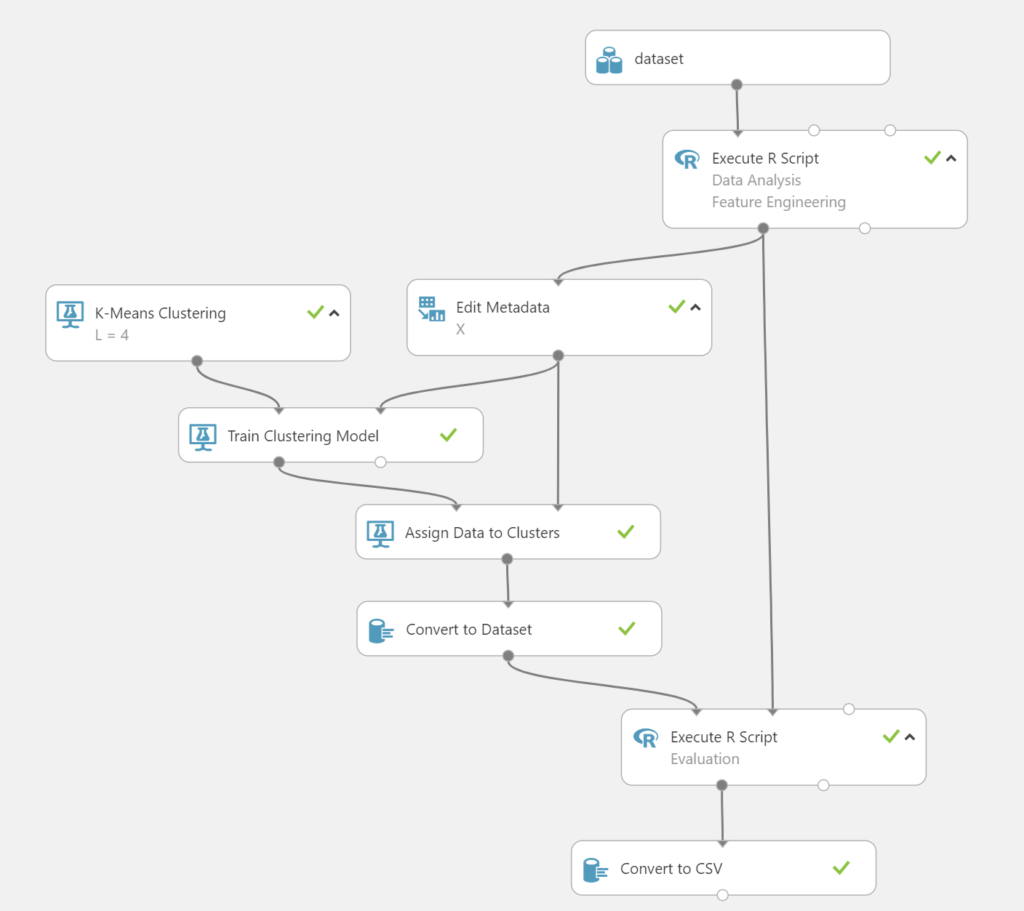# K-Means Clustering at Work

## Summary

This article presents my analysis of an unsupervised learning experiment on a dataset that contains clusters. The goal of the experiment is to find the cluster model. The experiment is based on $$7,500$$ observations and initially $$23$$ features (including $$1$$ categorical feature).

After analyzing the dataset using principal component analysis, selection of the features was applied. The remaining number of features was $$16$$.

The train clustering model was created after the feature engineering step. I excluded the categorical feature for evaluation purposes.

Finally, I evaluated the model by comparing the cluster and categorical value for each observation. The evaluation showed an average Silhouette coefficient of $$0.7$$. So, I may conclude that my clustering configuration is appropriate.

## References

 Wikipedia, K-Means Clustering

 Wikipedia, Silhouette Coefficient

## Nomenclature

\begin{array}{|l|l|}
\hline
\textbf{Symbol} & \textbf{Definition} \\
\hline
\mathbb{R} & \text{Set of real numbers}\\
\mu(\cdot) & \text{Mean}\\||\cdot||_{2} &
\text{Euclidean norm}\\
C & \text{Set of categorical values}\\
S & \text{Set of clusters}\\
C_{k} & \text{The k-th categorical value in } C\\
S_{k} & \text{The k-th cluster in } S\\
L & \text{Number of clusters}\\
m & \text{Number of observations in the experiment}\\
n & \text{Number of features in the experiment}\\
X: \mathbb{R}^{n} \rightarrow \mathbb{R}^{m} & m \times n \text{ design matrix}\\
\mathbf{x_{k}} \in \mathbb{R}^{m}& \text{The k-th feature vector in } X\\
\mathbf{o} \in \mathbb{R}^{n}& \text{An observation in } S_{k}\\
\hline
\end{array}

## Dataset

My dataset consists of $$23$$ features and $$7,500$$ observations. The dataset contains $$22$$ numerical features and $$1$$ categorical feature. The numerical features contains random noise. The values of the categorical feature belong to the set $$\{C_{0}, C_{1}, C_{2},C_{3}\}$$.

## Data Analysis

The structure of the data points is obtained from principal component analysis: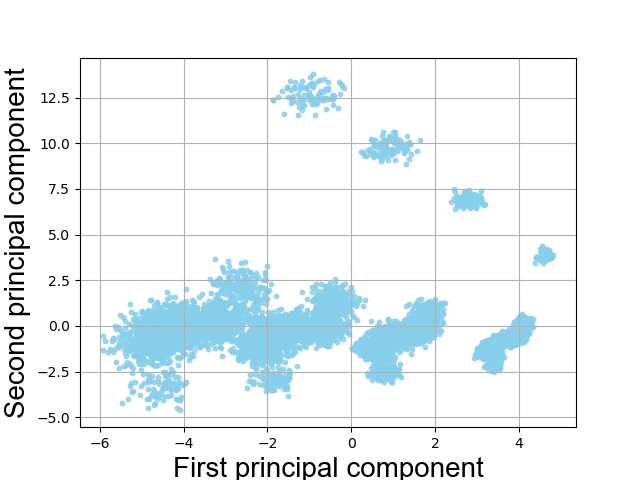The figure shows clusters, but perhaps also outliers. That brings me to the next step.

## Feature Engineering

Since I was expecting $$4$$ clusters for this experiment, I have removed $$7$$ numerical features by trial and error. The figure below show you the result.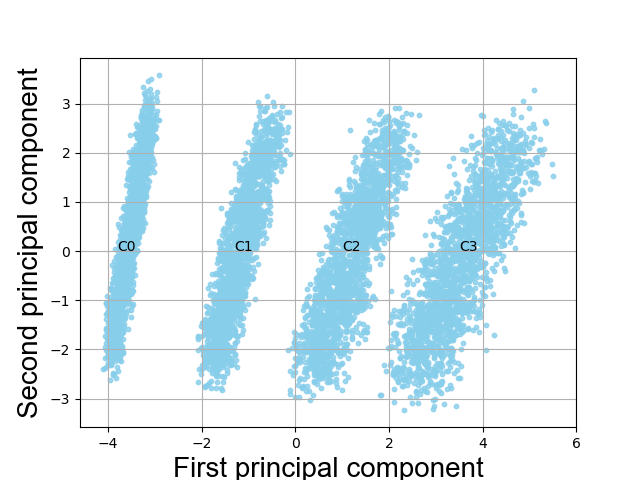The distribution of the observations over the categorical values is shown in the table below.
\begin{array}{|c|c|}
\hline
\textbf{Categorical value}&\textbf{Frequency}\\
\hline
C_{0} & 1903 \\
C_{1} & 1896\\
C_{2} & 1874\\
C_{3} & 1827\\\hline
\end{array}

## Design Matrix

The design matrix $$X$$ follows from the feature engineering step with $$m = 7500$$ observations and $$n=15$$ variables:
$X = \begin{bmatrix} \mathbf{x_{1}}&\mathbf{x_{2}}&\cdots&\mathbf{x_{15}} \end{bmatrix}.$
The categorical values are excluded for evaluation purposes.

## K-Means Clustering

The goal of the algorithm is to find the $$L = 4$$ clusters and each observation belongs to the cluster with the nearest mean. The algorithm solves the following optimization problem :
$\arg \min_{S} \sum^{L}_{k = 1}\sum_{\mathbf{o} \in S_{k}} \parallel \mathbf{o} – \mu(S_{i})\parallel_{2}^{2}.$

The inputs of the algorithm are $$L = 4$$ and the design matrix $$X$$.

The output concerns a cluster centroid subspace spanned by the principal directions.

## Model

After running the algorithm, a train clustering model is created. The figure below show you the result.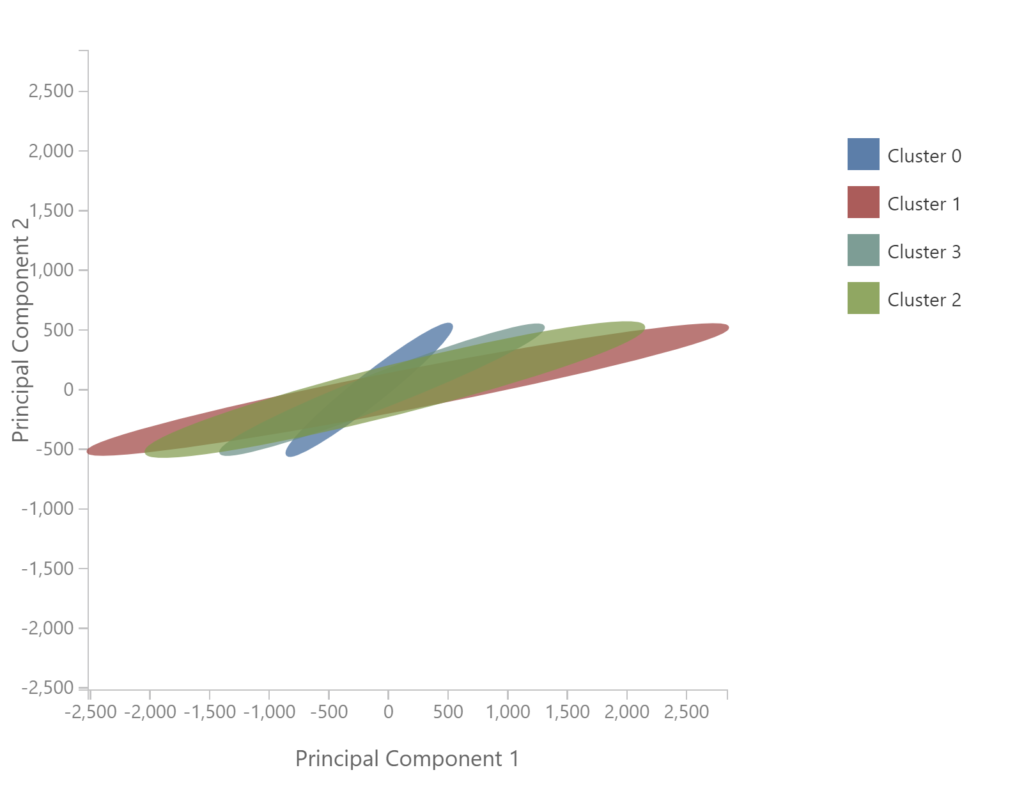## Evaluation

I evaluated the model by comparing the cluster and categorical value for each observation. In the figure below you can see the result.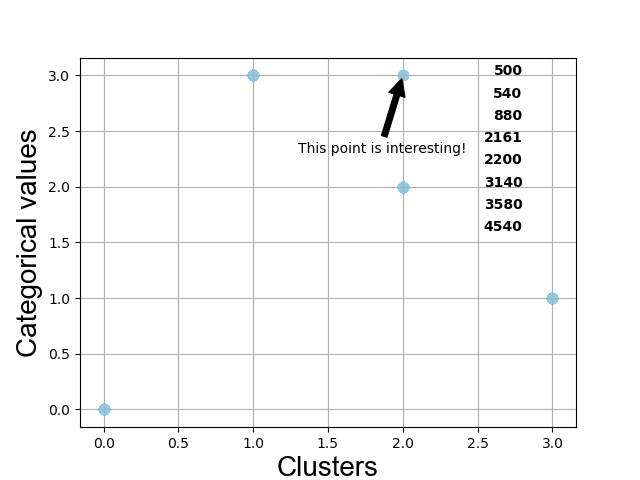The figure show you $$5$$ points in stead of $$4$$ points contrary to expectations. The point of interest concerns $$8$$ observations. The observations are assigned to the categorical value $$C_{3}$$ as shown in the figure below.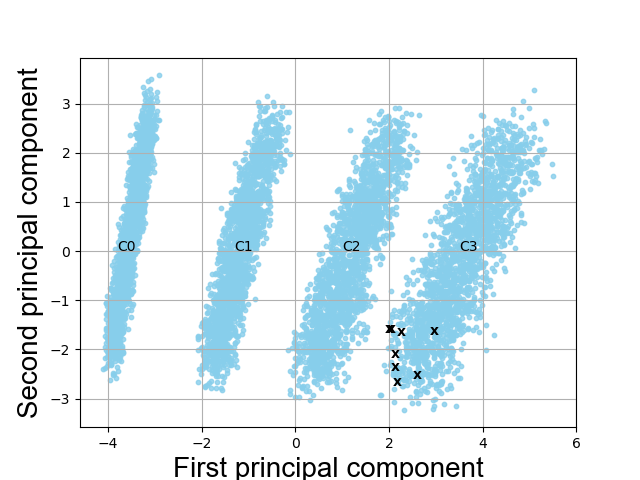Thus the accuracy of the trained model is $$\left(100 – \frac{800}{7500}\right)\% = 99.89\%$$.

The Sihouette coefficient is a measure of how similar a data point is to its own cluster (cohesion) compared to other clusters (separation) . Since the clusters have a Silhouette value $$> 0.5$$, I may conclude that my clustering configuration is appropriate: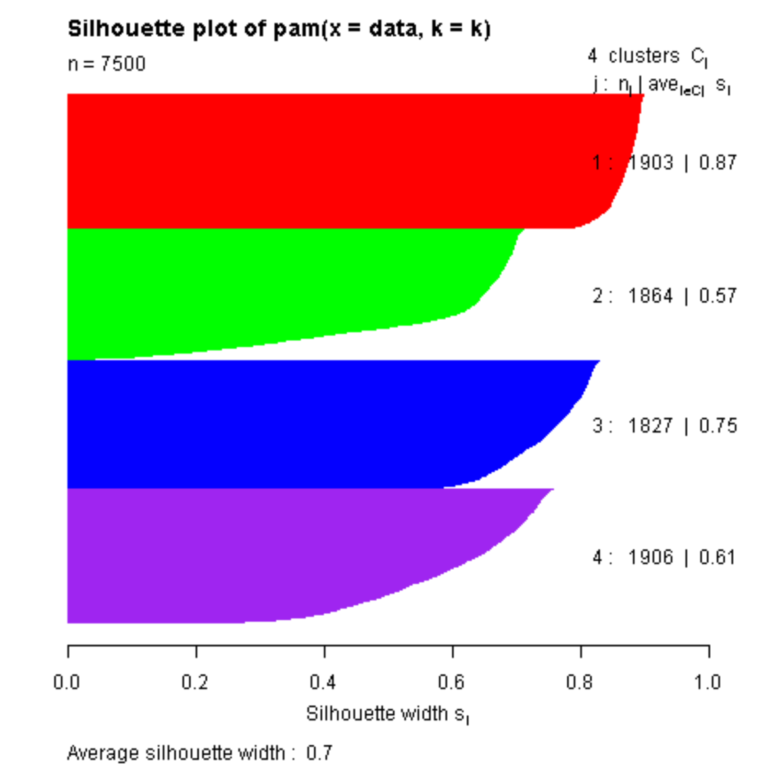## Conclusion

The experiment was performed on a complex dataset that contains clusters, but perhaps also outliers. After the process of feature engineering the observations are clearly separated in clusters. This analysis has shown that the $$4$$ clusters can be found by training the k-means clustering model. The evaluation showed an average Silhouette coefficient of $$0.7$$.

## Machine Learning Experiment